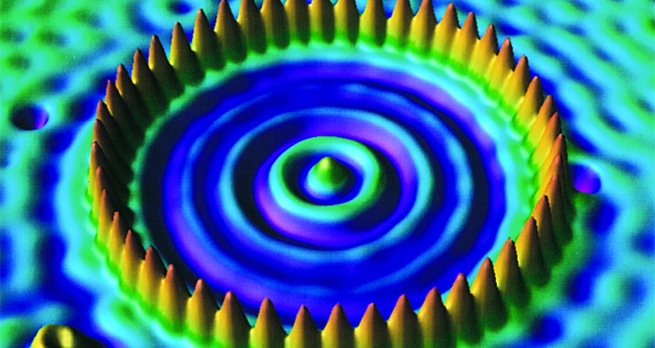Collisions and conservation laws

Start this free course now. Just create an account and sign in. Enrol and complete the course for a free statement of participation or digital badge if available.

Free course

# 3.1 Elastic collisions with a stationary target

We begin with an example of a one-dimensional elastic collision between two particles of identical mass, one of which is initially stationary. Our aim now is to find the final velocity of each particle after the collision.

## Activity 1

A particle of mass moves along the -axis with velocity and collides elastically with an identical particle at rest. What are the velocities of the two particles after the collision?

Let the final velocities be and . Conservation of momentum along the -axis gives

Equation label: (3)

and conservation of kinetic energy for this elastic collision gives

Equation label: (4)

By eliminating common factors, Equation 3 can be simplified to give

Equation label: (5)

and Equation 4 can be treated similarly to give

Equation label: (6)

Rearranging Equation 6 gives

the right-hand side of which may be rewritten using the general identity , thus

Dividing both sides of this last equation by ,

and using Equation 5 to simplify the resulting right-hand side gives

Comparing this expression for with that in Equation 5 shows that

The result of Example 1 will be familiar to anyone who has seen the head-on collision of two bowls on a bowling green. The moving one stops, and the one that was initially stationary moves off with the original velocity of the first. In effect, the bowls exchange velocities.

## Activity 2

Predict qualitatively (i.e. without calculation) what would happen when a body of mass collides with another body of mass that is initially at rest if:

(a) (The symbol should be read as ‘is very much greater than’.)

Experience should tell you that a high-mass projectile fired at a low-mass target would be essentially unaffected by the collision.

(b) .

A low-mass projectile fired at a massive target would bounce back with unchanged speed.

S217_1